# RD Sharma Solutions For Class 12 Maths Exercise 2.3 Chapter 2 Function

RD Sharma Solutions for Class 12 Maths Exercise 2.3 Chapter 2 Function consists of problems on finding the composition of real functions. Class 12 is a major turning point in students’ lives. It primarily gears them up to make critical decisions regarding their further studies and future goals. These problems are solved in a simple way to speed up the exam preparation of students. It mainly helps during revision and improves confidence among students before appearing for the board exam. To gain better knowledge about the concepts explained in this exercise, they can refer RD Sharma Solutions Class 12 Maths Chapter 2 Functions Exercise 2.3 free PDF, which are given below.

## Download the PDF of RD Sharma Solutions For Class 12 Chapter 2 – Function Exercise 2.3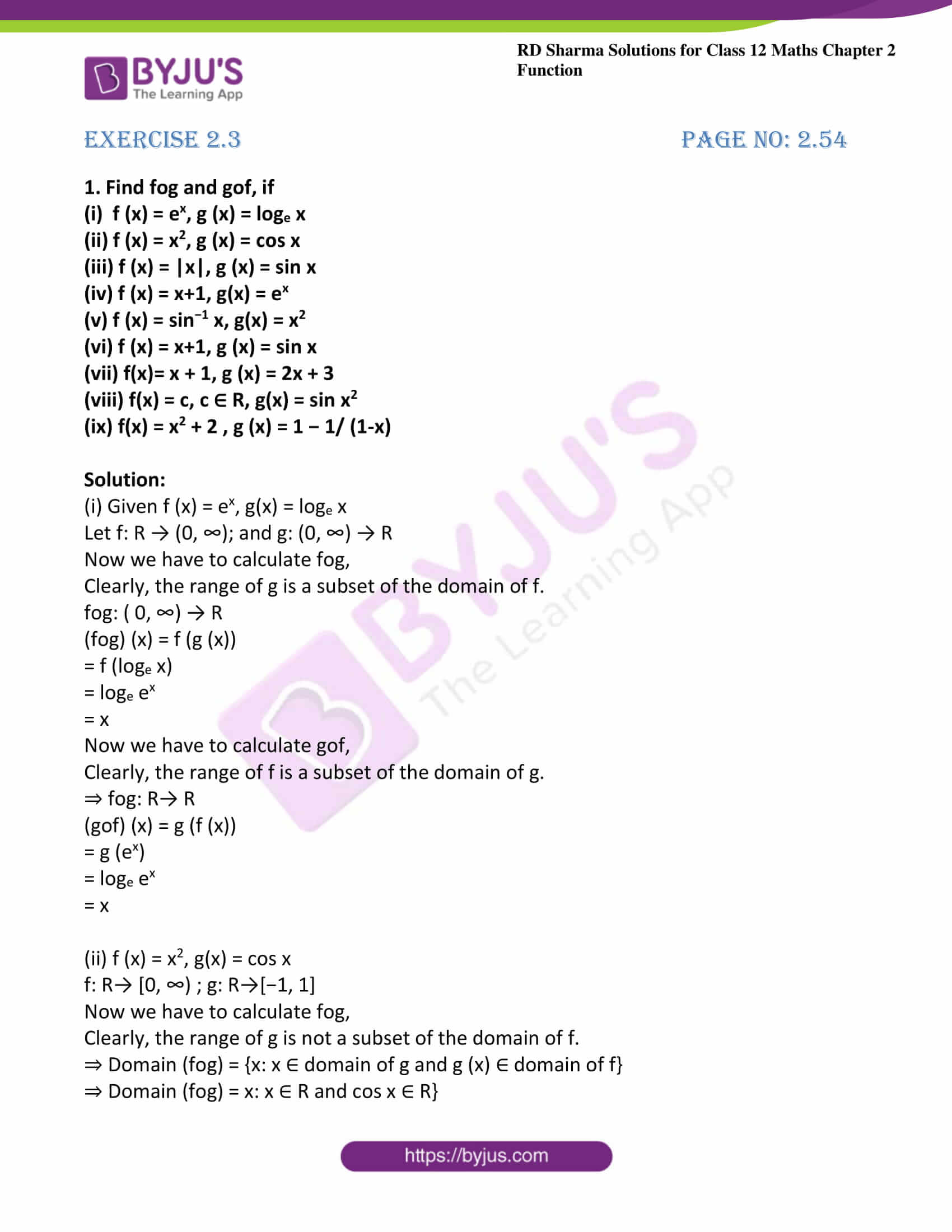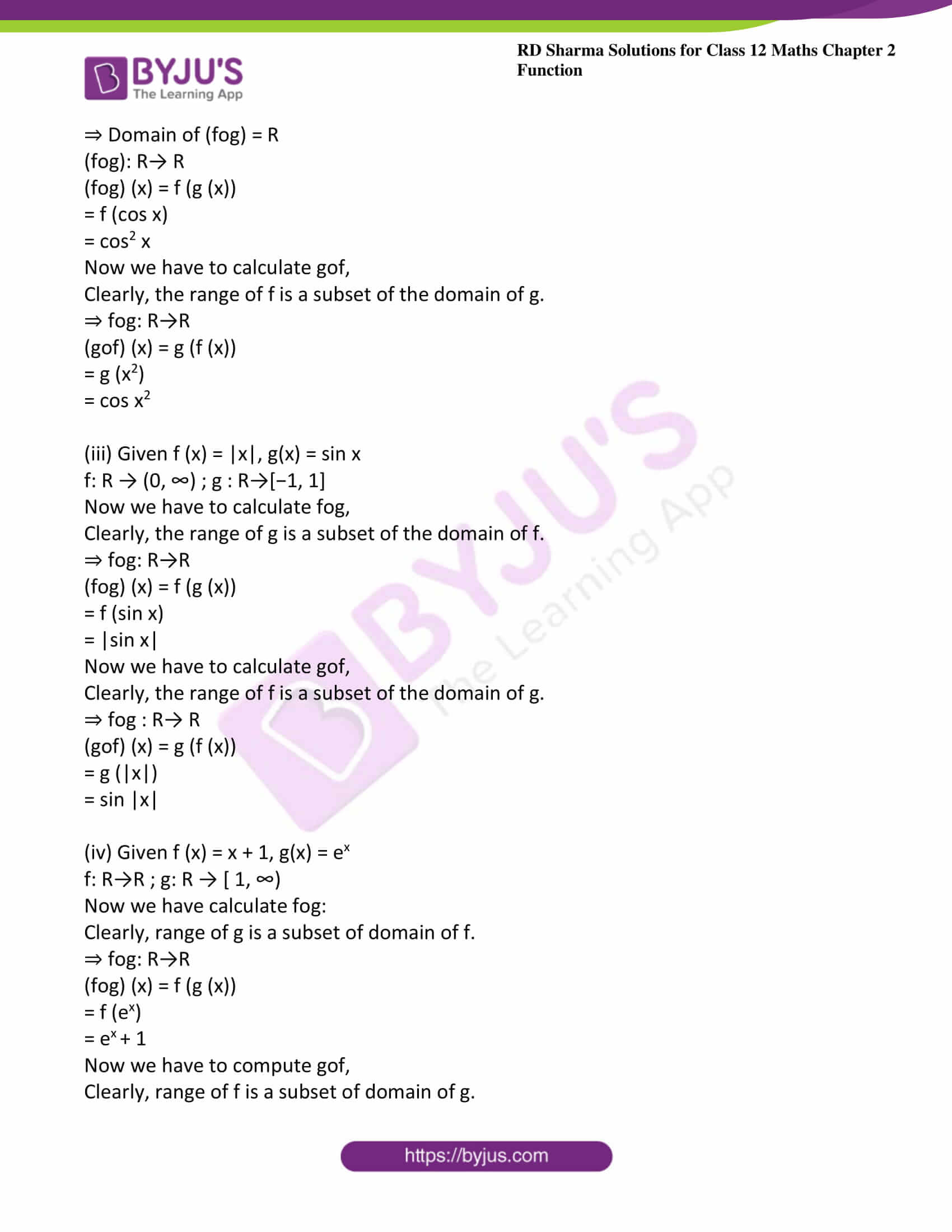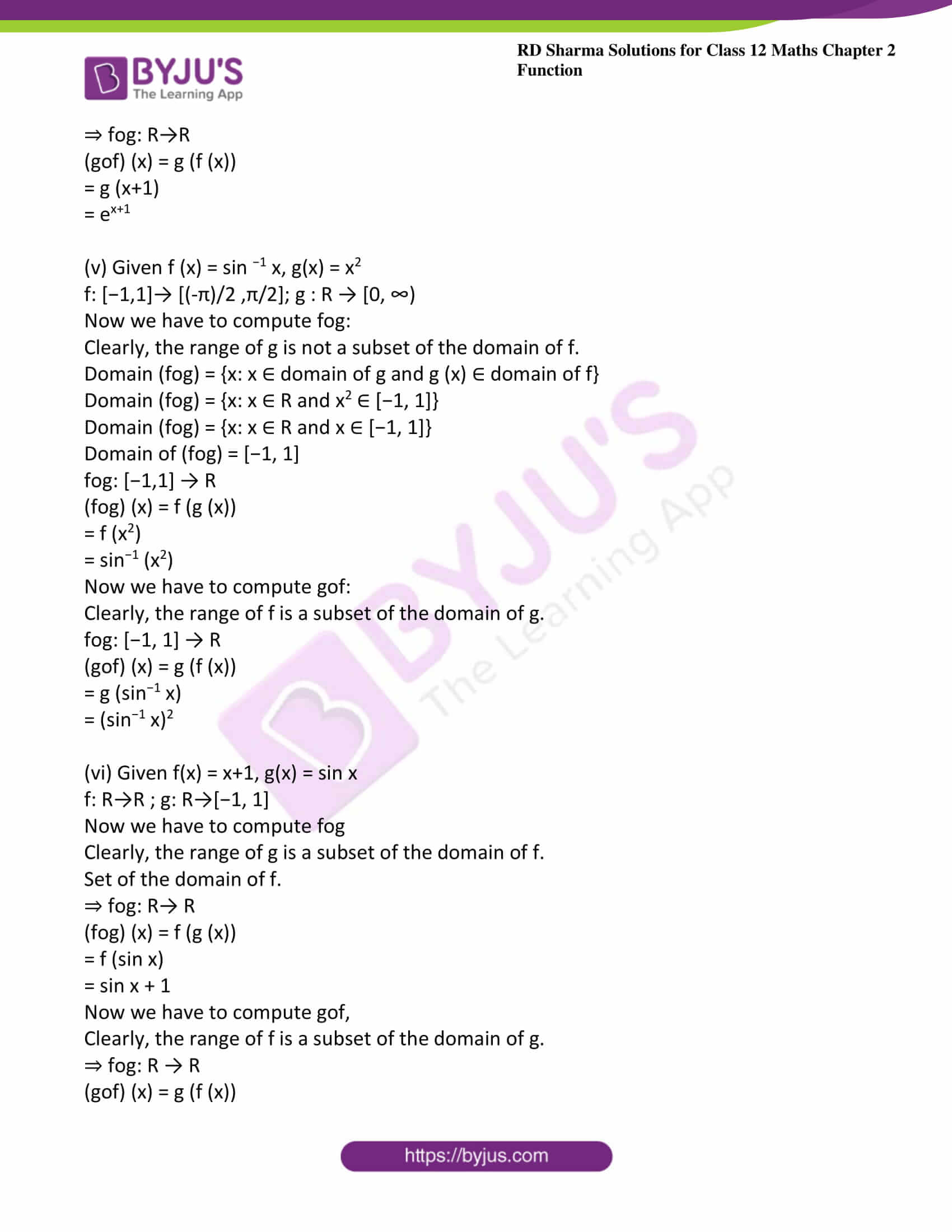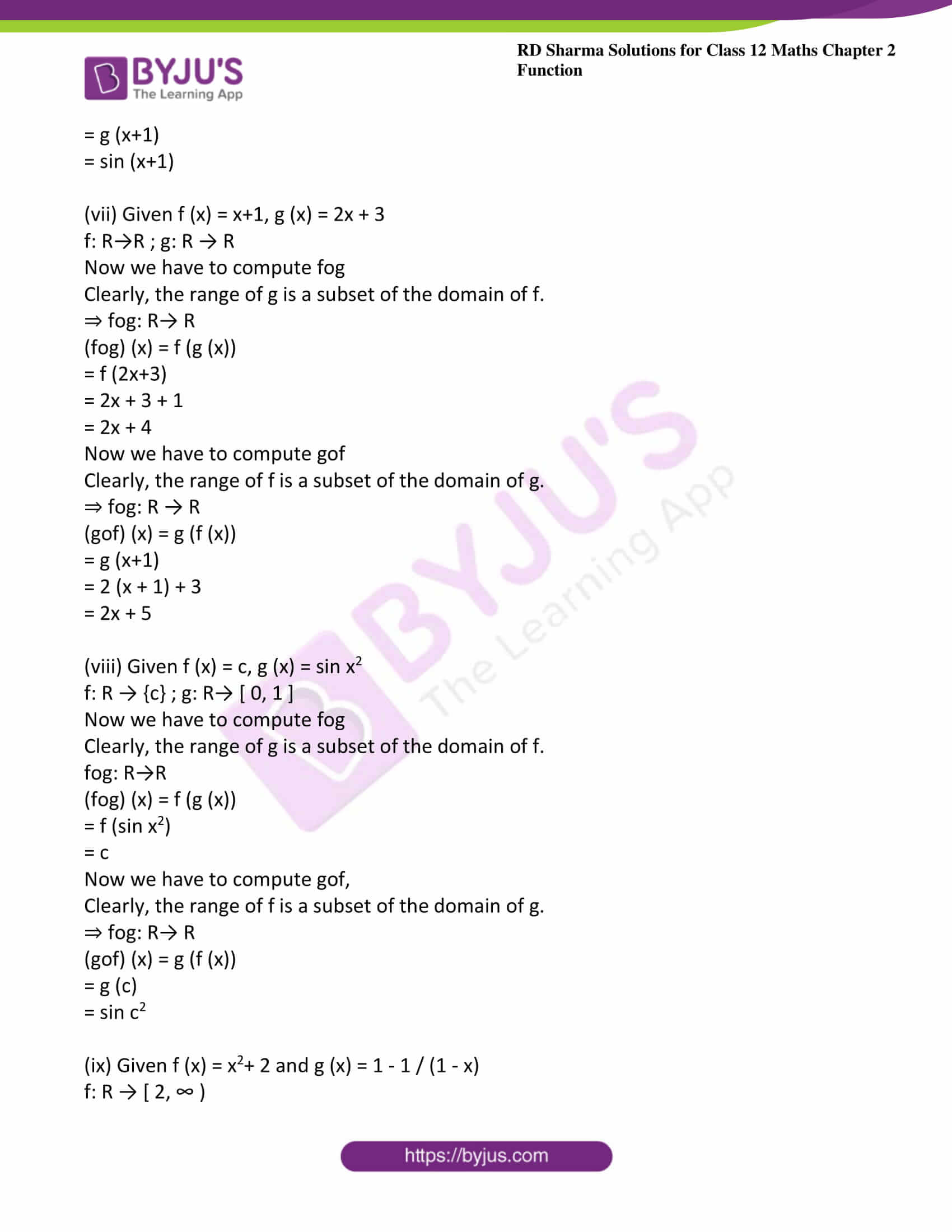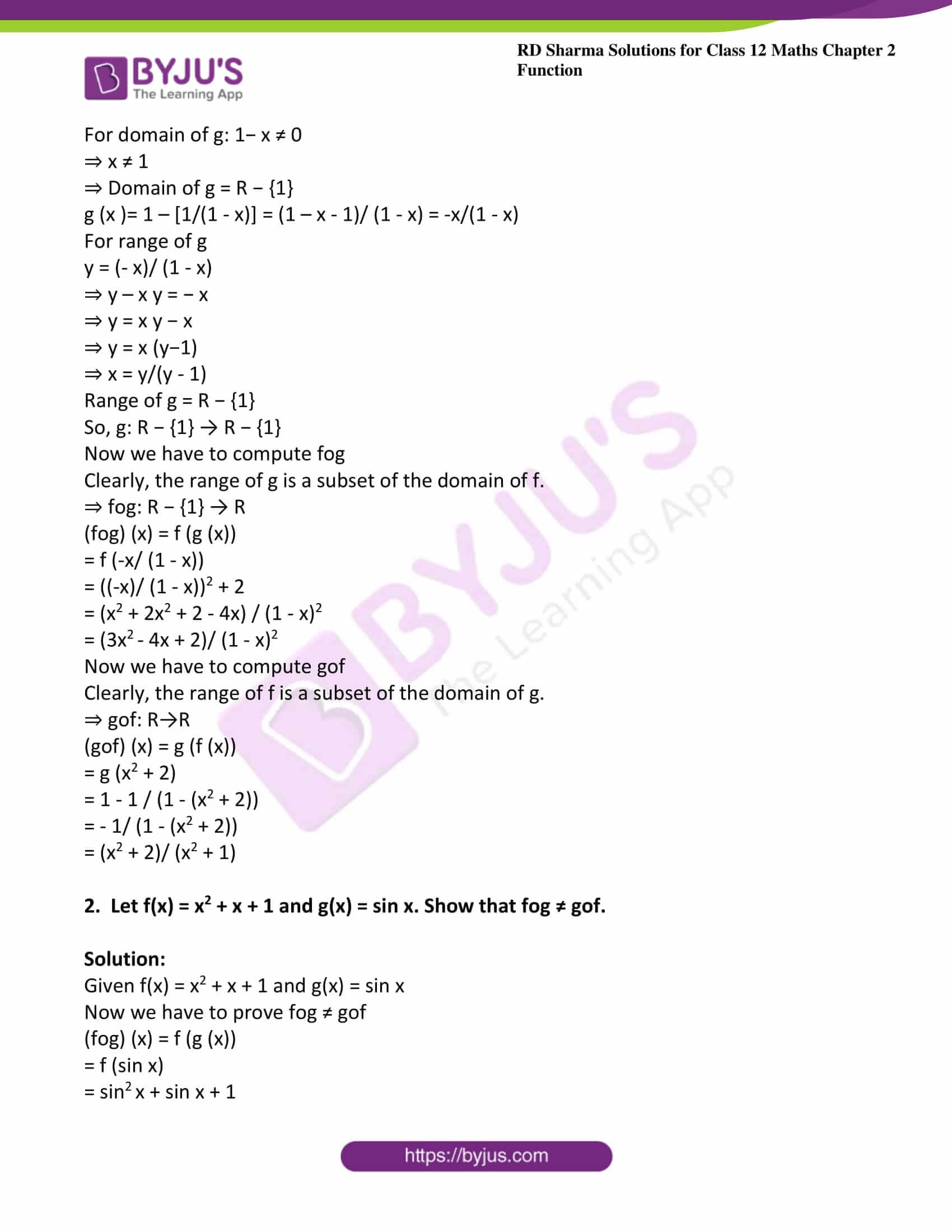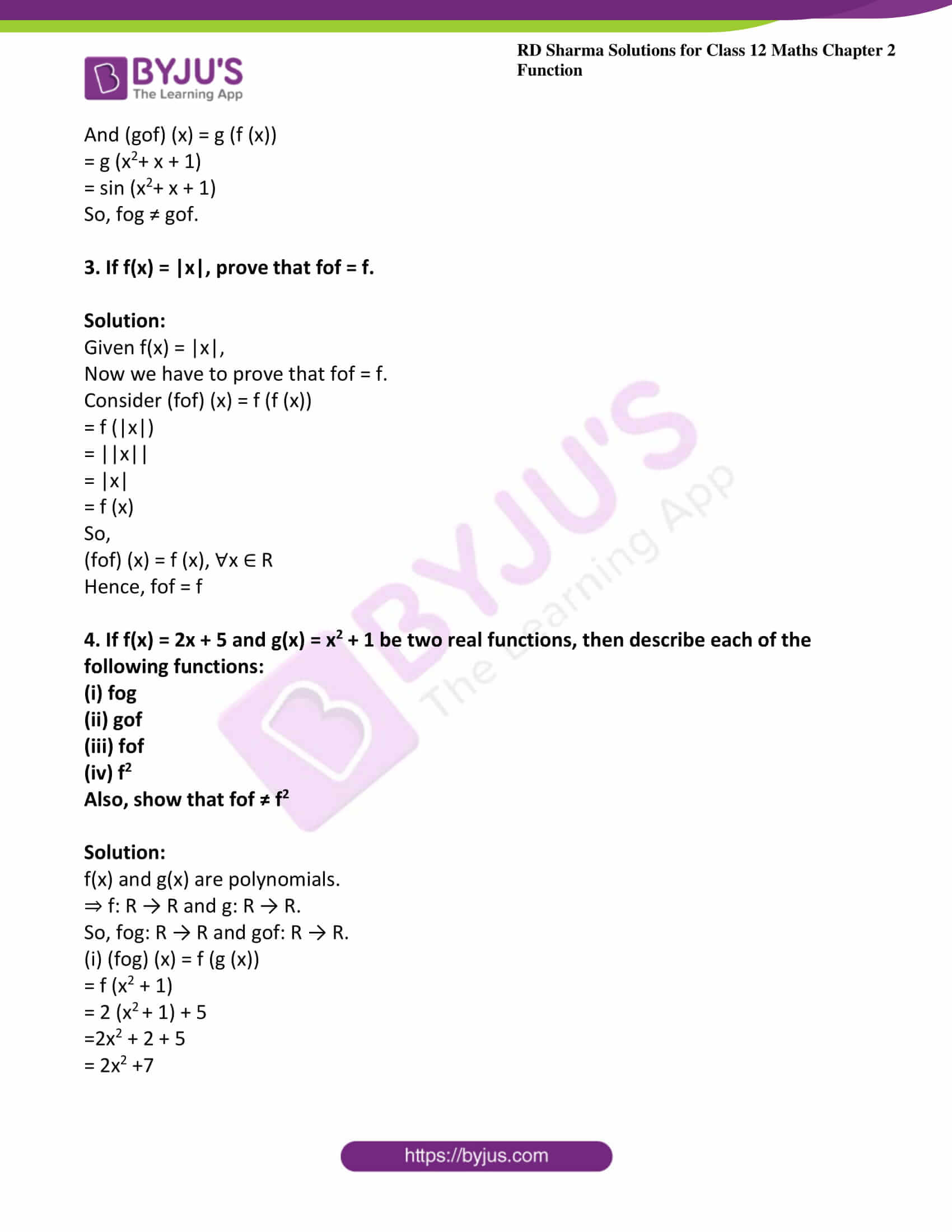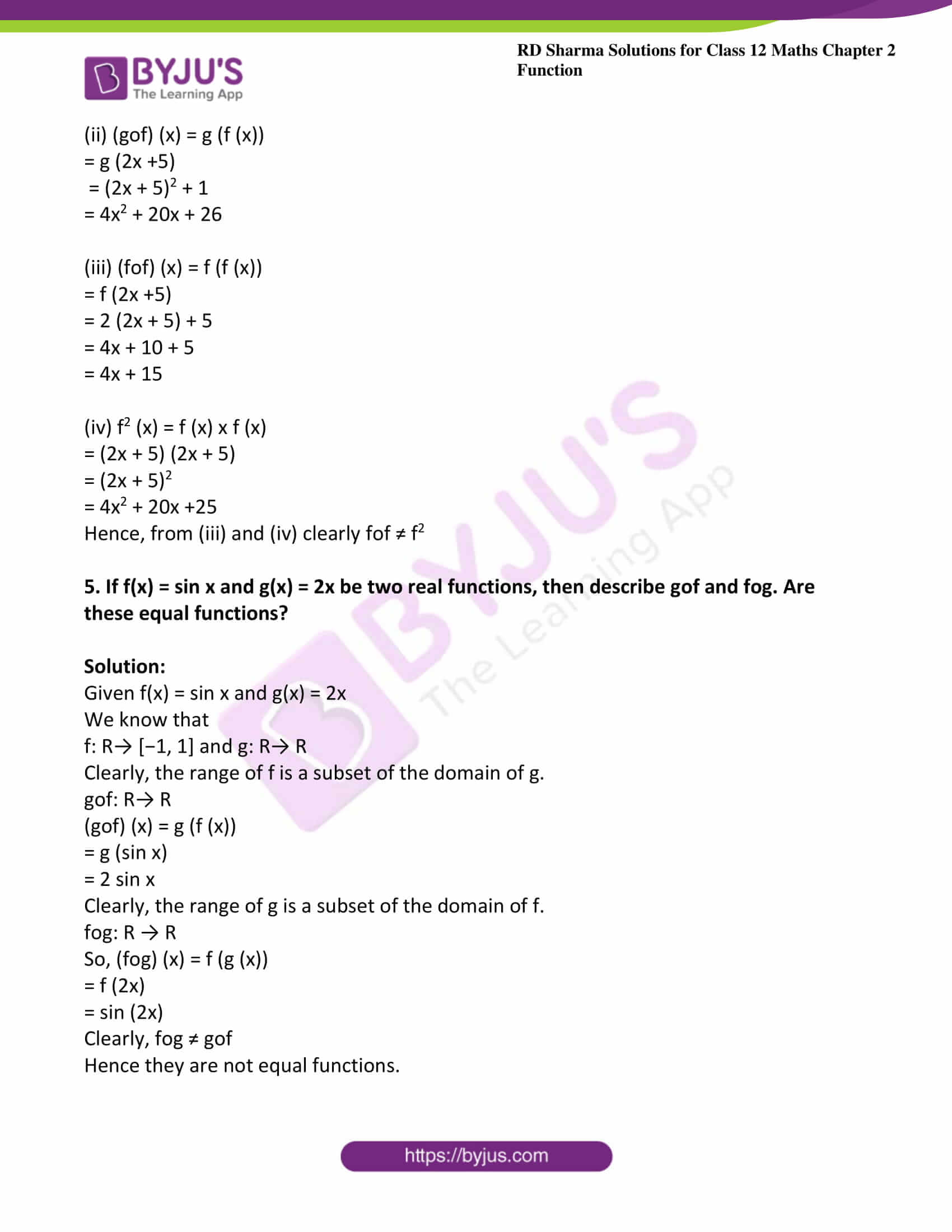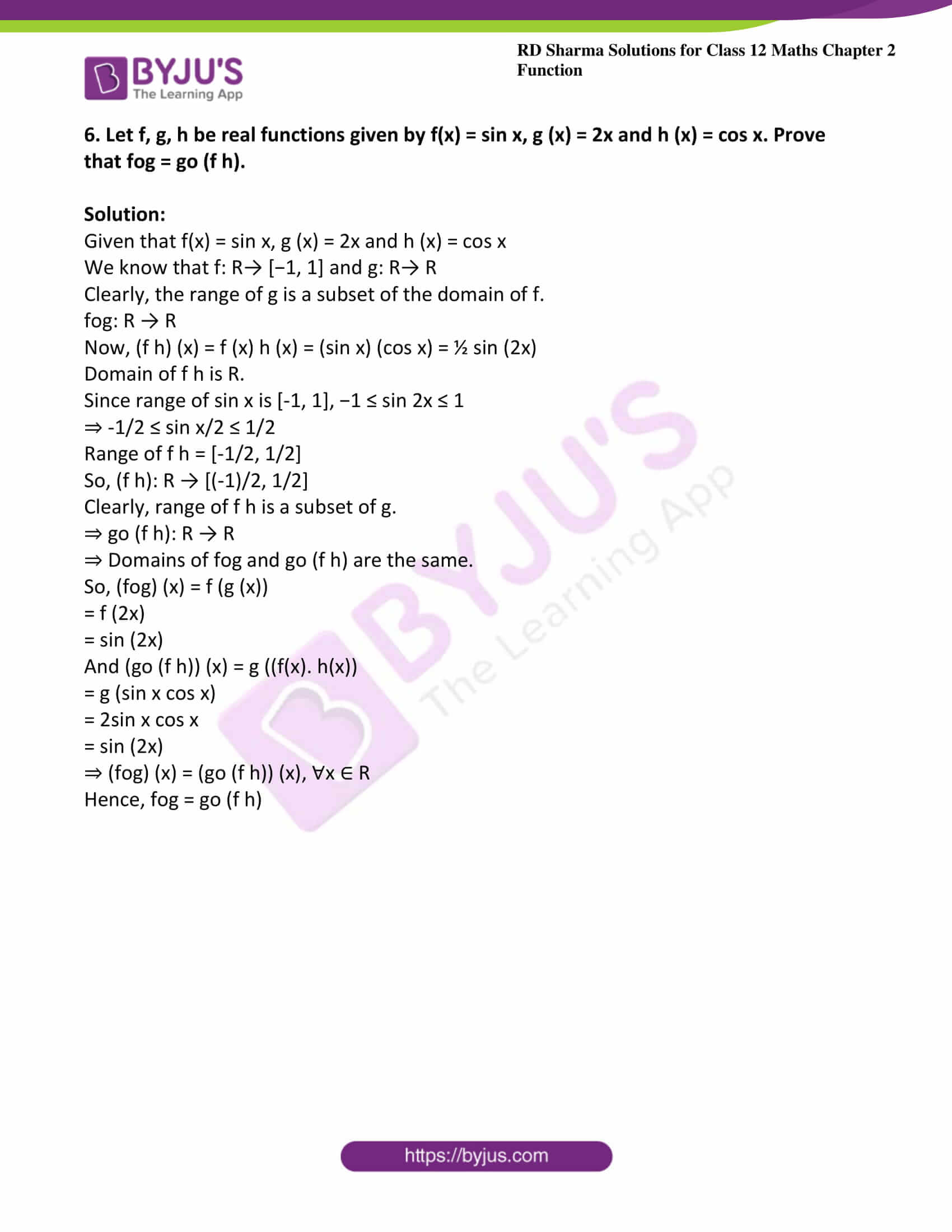### Access other exercises of RD Sharma Solutions For Class 12 Chapter 2 – Function

Exercise 2.1 Solutions

Exercise 2.2 Solutions

Exercise 2.4 Solutions

### Exercise 2.3 Page No: 2.54

1. Find fog and gof, if

(i)  f (x) = ex, g (x) = loge x

(ii) f (x) = x2, g (x) = cos x

(iii) f (x) = |x|, g (x) = sin x

(iv) f (x) = x+1, g(x) = ex

(v) f (x) = sin−1 x, g(x) = x2

(vi) f (x) = x+1, g (x) = sin x

(vii) f(x)= x + 1, g (x) = 2x + 3

(viii) f(x) = c, c ∈ R, g(x) = sin x2

(ix) f(x) = x2 + 2 , g (x) = 1 − 1/ (1-x)

Solution:

(i) Given f (x) = ex, g(x) = loge x

Let f: R → (0, ∞); and g: (0, ∞) → R

Now we have to calculate fog,

Clearly, the range of g is a subset of the domain of f.

fog: ( 0, ∞) → R

(fog) (x) = f (g (x))

= f (loge x)

= loge ex

= x

Now we have to calculate gof,

Clearly, the range of f is a subset of the domain of g.

⇒ fog: R→ R

(gof) (x) = g (f (x))

= g (ex)

= loge ex

= x

(ii) f (x) = x2, g(x) = cos x

f: R→ [0, ∞) ; g: R→[−1, 1]

Now we have to calculate fog,

Clearly, the range of g is not a subset of the domain of f.

⇒ Domain (fog) = {x: x ∈ domain of g and g (x) ∈ domain of f}

⇒ Domain (fog) = x: x ∈ R and cos x ∈ R}

⇒ Domain of (fog) = R

(fog): R→ R

(fog) (x) = f (g (x))

= f (cos x)

= cos2 x

Now we have to calculate gof,

Clearly, the range of f is a subset of the domain of g.

⇒ fog: R→R

(gof) (x) = g (f (x))

= g (x2)

= cos x2

(iii) Given f (x) = |x|, g(x) = sin x

f: R → (0, ∞) ; g : R→[−1, 1]

Now we have to calculate fog,

Clearly, the range of g is a subset of the domain of f.

⇒ fog: R→R

(fog) (x) = f (g (x))

= f (sin x)

= |sin x|

Now we have to calculate gof,

Clearly, the range of f is a subset of the domain of g.

⇒ fog : R→ R

(gof) (x) = g (f (x))

= g (|x|)

= sin |x|

(iv) Given f (x) = x + 1, g(x) = ex

f: R→R ; g: R → [ 1, ∞)

Now we have calculate fog:

Clearly, range of g is a subset of domain of f.

⇒ fog: R→R

(fog) (x) = f (g (x))

= f (ex)

= ex + 1

Now we have to compute gof,

Clearly, range of f is a subset of domain of g.

⇒ fog: R→R

(gof) (x) = g (f (x))

= g (x+1)

= ex+1

(v) Given f (x) = sin −1 x, g(x) = x2

f: [−1,1]→ [(-π)/2 ,π/2]; g : R → [0, ∞)

Now we have to compute fog:

Clearly, the range of g is not a subset of the domain of f.

Domain (fog) = {x: x ∈ domain of g and g (x) ∈ domain of f}

Domain (fog) = {x: x ∈ R and x2 ∈ [−1, 1]}

Domain (fog) = {x: x ∈ R and x ∈ [−1, 1]}

Domain of (fog) = [−1, 1]

fog: [−1,1] → R

(fog) (x) = f (g (x))

= f (x2)

= sin−1 (x2)

Now we have to compute gof:

Clearly, the range of f is a subset of the domain of g.

fog: [−1, 1] → R

(gof) (x) = g (f (x))

= g (sin−1 x)

= (sin−1 x)2

(vi) Given f(x) = x+1, g(x) = sin x

f: R→R ; g: R→[−1, 1]

Now we have to compute fog

Clearly, the range of g is a subset of the domain of f.

Set of the domain of f.

⇒ fog: R→ R

(fog) (x) = f (g (x))

= f (sin x)

= sin x + 1

Now we have to compute gof,

Clearly, the range of f is a subset of the domain of g.

⇒ fog: R → R

(gof) (x) = g (f (x))

= g (x+1)

= sin (x+1)

(vii) Given f (x) = x+1, g (x) = 2x + 3

f: R→R ; g: R → R

Now we have to compute fog

Clearly, the range of g is a subset of the domain of f.

⇒ fog: R→ R

(fog) (x) = f (g (x))

= f (2x+3)

= 2x + 3 + 1

= 2x + 4

Now we have to compute gof

Clearly, the range of f is a subset of the domain of g.

⇒ fog: R → R

(gof) (x) = g (f (x))

= g (x+1)

= 2 (x + 1) + 3

= 2x + 5

(viii) Given f (x) = c, g (x) = sin x2

f: R → {c} ; g: R→ [ 0, 1 ]

Now we have to compute fog

Clearly, the range of g is a subset of the domain of f.

fog: R→R

(fog) (x) = f (g (x))

= f (sin x2)

= c

Now we have to compute gof,

Clearly, the range of f is a subset of the domain of g.

⇒ fog: R→ R

(gof) (x) = g (f (x))

= g (c)

= sin c2

(ix) Given f (x) = x2+ 2 and g (x) = 1 – 1 / (1 – x)

f: R → [ 2, ∞ )

For domain of g: 1− x ≠ 0

⇒ x ≠ 1

⇒ Domain of g = R − {1}

g (x )= 1 – [1/(1 – x)] = (1 – x – 1)/ (1 – x) = -x/(1 – x)

For range of g

y = (- x)/ (1 – x)

⇒ y – x y = − x

⇒ y = x y − x

⇒ y = x (y−1)

⇒ x = y/(y – 1)

Range of g = R − {1}

So, g: R − {1} → R − {1}

Now we have to compute fog

Clearly, the range of g is a subset of the domain of f.

⇒ fog: R − {1} → R

(fog) (x) = f (g (x))

= f (-x/ (1 – x))

= ((-x)/ (1 – x))2 + 2

= (x2 + 2x2 + 2 – 4x) / (1 – x)2

= (3x2 – 4x + 2)/ (1 – x)2

Now we have to compute gof

Clearly, the range of f is a subset of the domain of g.

⇒ gof: R→R

(gof) (x) = g (f (x))

= g (x2 + 2)

= 1 – 1 / (1 – (x2 + 2))

= – 1/ (1 – (x2 + 2))

= (x2 + 2)/ (x2 + 1)

2.  Let f(x) = x2 + x + 1 and g(x) = sin x. Show that fog ≠ gof.

Solution:

Given f(x) = x2 + x + 1 and g(x) = sin x

Now we have to prove fog ≠ gof

(fog) (x) = f (g (x))

= f (sin x)

= sinx + sin x + 1

And (gof) (x) = g (f (x))

= g (x2+ x + 1)

= sin (x2+ x + 1)

So, fog ≠ gof.

3. If f(x) = |x|, prove that fof = f.

Solution:

Given f(x) = |x|,

Now we have to prove that fof = f.

Consider (fof) (x) = f (f (x))

= f (|x|)

= ||x||

= |x|

= f (x)

So,

(fof) (x) = f (x), ∀x ∈ R

Hence, fof = f

4. If f(x) = 2x + 5 and g(x) = x2 + 1 be two real functions, then describe each of the following functions:
(i) fog
(ii) gof
(iii) fof
(iv) f2
Also, show that fof ≠ f2

Solution:

f(x) and g(x) are polynomials.

⇒ f: R → R and g: R → R.

So, fog: R → R and gof: R → R.

(i) (fog) (x) = f (g (x))

= f (x2 + 1)

= 2 (x2 + 1) + 5

=2x2 + 2 + 5

= 2x2 +7

(ii) (gof) (x) = g (f (x))

= g (2x +5)

= (2x + 5)2 + 1

= 4x2 + 20x + 26

(iii) (fof) (x) = f (f (x))

= f (2x +5)

= 2 (2x + 5) + 5

= 4x + 10 + 5

= 4x + 15

(iv) f2 (x) = f (x) x f (x)

= (2x + 5) (2x + 5)

= (2x + 5)2

= 4x2 + 20x +25

Hence, from (iii) and (iv) clearly fof ≠ f2

5. If f(x) = sin x and g(x) = 2x be two real functions, then describe gof and fog. Are these equal functions?

Solution:

Given f(x) = sin x and g(x) = 2x

We know that

f: R→ [−1, 1] and g: R→ R

Clearly, the range of f is a subset of the domain of g.

gof: R→ R

(gof) (x) = g (f (x))

= g (sin x)

= 2 sin x

Clearly, the range of g is a subset of the domain of f.

fog: R → R

So, (fog) (x) = f (g (x))

= f (2x)

= sin (2x)

Clearly, fog ≠ gof

Hence they are not equal functions.

6. Let f, g, h be real functions given by f(x) = sin x, g (x) = 2x and h (x) = cos x. Prove that fog = go (f h).

Solution:

Given that f(x) = sin x, g (x) = 2x and h (x) = cos x

We know that f: R→ [−1, 1] and g: R→ R

Clearly, the range of g is a subset of the domain of f.

fog: R → R

Now, (f h) (x) = f (x) h (x) = (sin x) (cos x) = ½ sin (2x)

Domain of f h is R.

Since range of sin x is [-1, 1], −1 ≤ sin 2x ≤ 1

⇒ -1/2 ≤ sin x/2 ≤ 1/2

Range of f h = [-1/2, 1/2]

So, (f h): R → [(-1)/2, 1/2]

Clearly, range of f h is a subset of g.

⇒ go (f h): R → R

⇒ Domains of fog and go (f h) are the same.

So, (fog) (x) = f (g (x))

= f (2x)

= sin (2x)

And (go (f h)) (x) = g ((f(x). h(x))

= g (sin x cos x)

= 2sin x cos x

= sin (2x)

⇒ (fog) (x) = (go (f h)) (x), ∀x ∈ R

Hence, fog = go (f h)